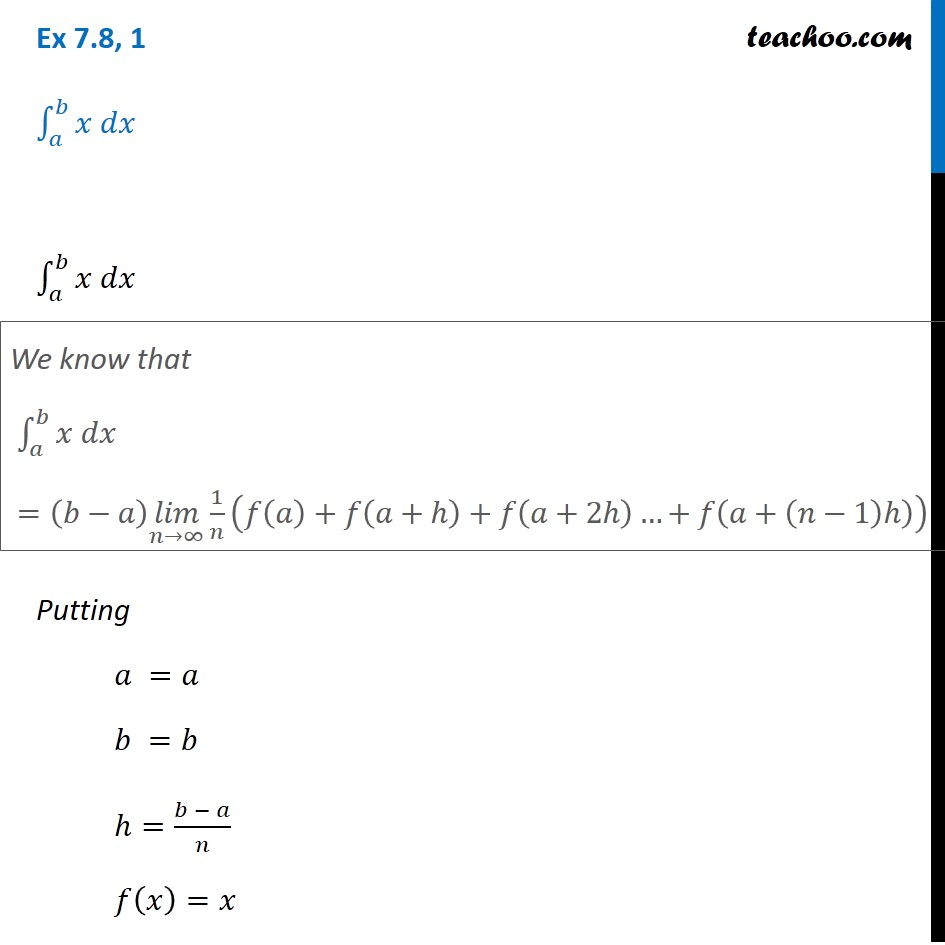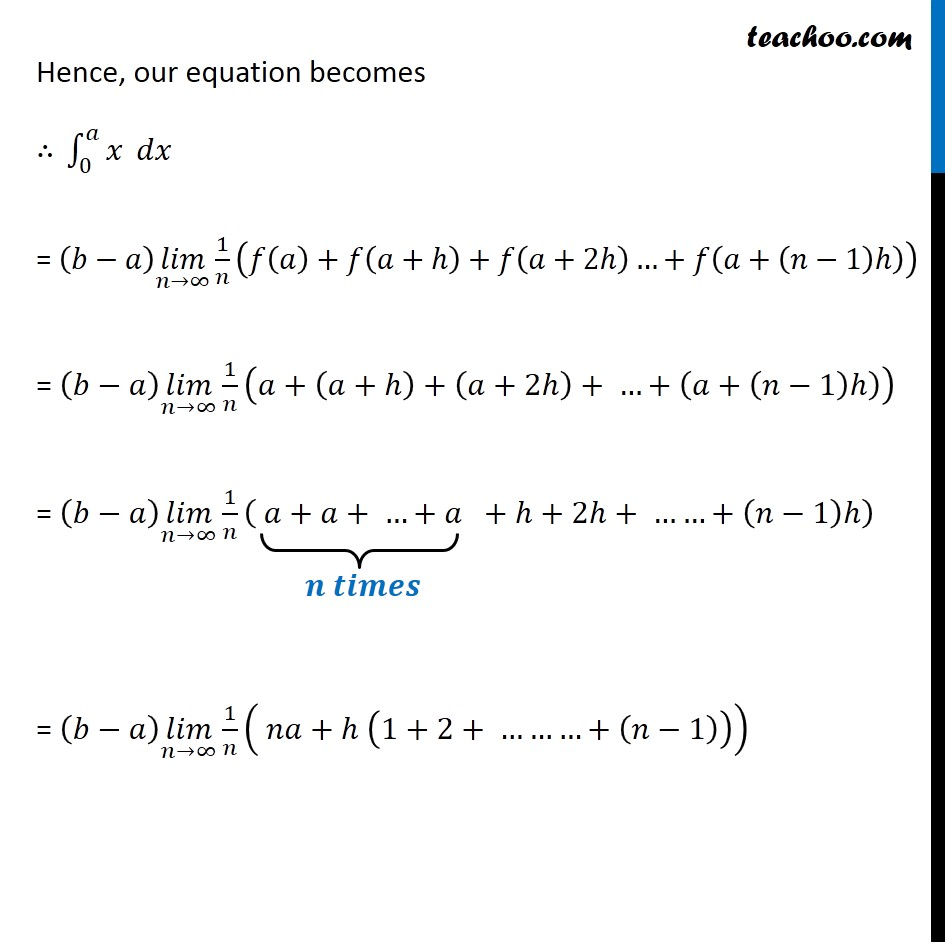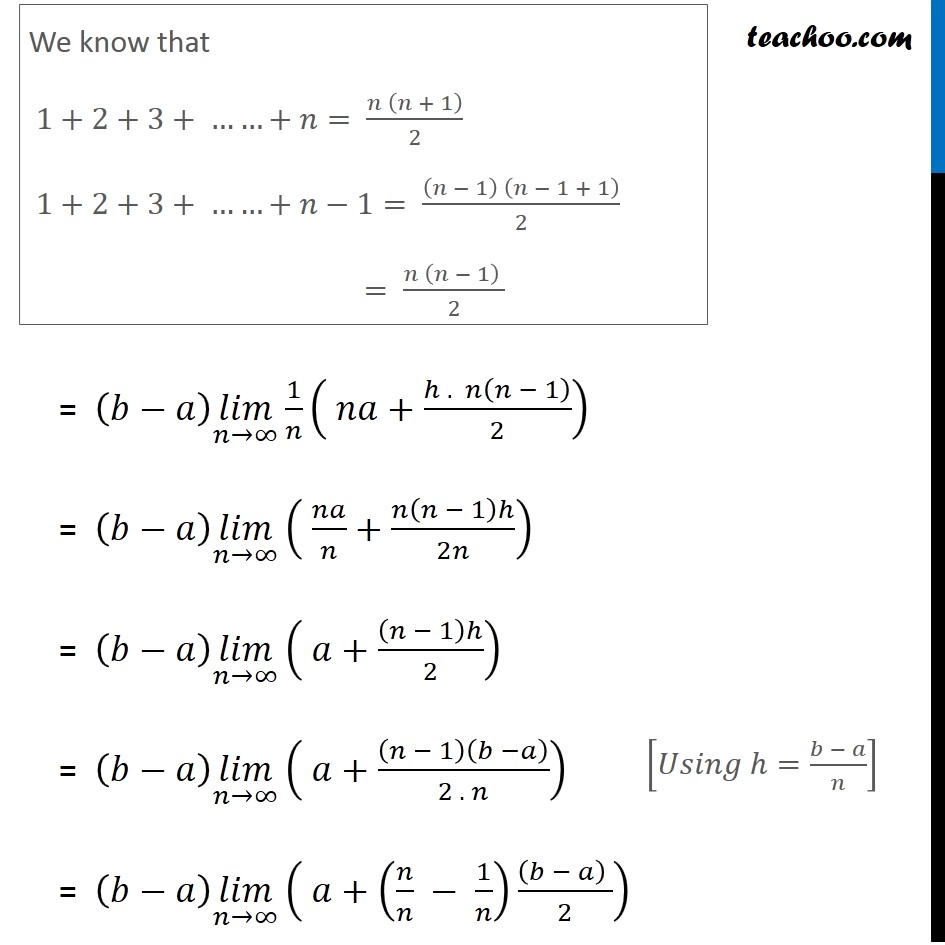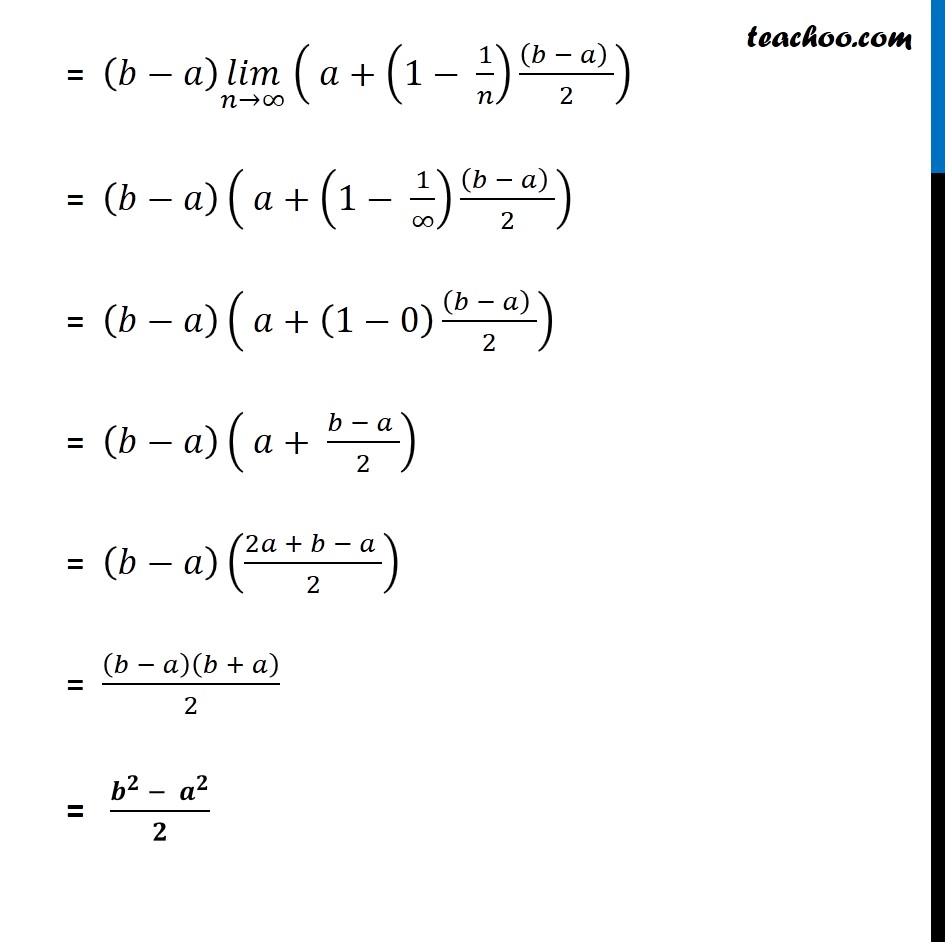1. Chapter 7 Class 12 Integrals (Term 2)
2. Concept wise
3. Definite Integral as a limit of a sum

Transcript

Ex 7.8, 1 ∫1_𝑎^𝑏▒〖𝑥 𝑑𝑥〗 ∫1_𝑎^𝑏▒〖𝑥 𝑑𝑥〗 Putting 𝑎 =𝑎 𝑏 =𝑏 ℎ=(𝑏 − 𝑎)/𝑛 𝑓(𝑥)=𝑥 Ex 7.8, 1 ∫1_𝑎^𝑏▒〖𝑥 𝑑𝑥〗 ∫1_𝑎^𝑏▒〖𝑥 𝑑𝑥〗 Putting 𝑎 =𝑎 𝑏 =𝑏 ℎ=(𝑏 − 𝑎)/𝑛 𝑓(𝑥)=𝑥 We know that ∫1_𝑎^𝑏▒〖𝑥 𝑑𝑥〗 =(𝑏−𝑎) (𝑙𝑖𝑚)┬(𝑛→∞) 1/𝑛 (𝑓(𝑎)+𝑓(𝑎+ℎ)+𝑓(𝑎+2ℎ)…+𝑓(𝑎+(𝑛−1)ℎ)) Hence we can write ∫1_𝑎^𝑏▒〖𝑥 𝑑𝑥〗 =(𝑏−𝑎) lim┬(n→∞) 1/𝑛 (𝑓(𝑎)+𝑓(𝑎+ℎ)+𝑓(𝑎+2ℎ)+… +𝑓(𝑎+(𝑛−1)ℎ) Here, 𝑓(𝑥)=𝑥 𝑓(𝑎)=𝑎 𝑓(𝑎+ℎ)=𝑎+ℎ 𝑓 (𝑎+2ℎ)=𝑎+2ℎ … 𝑓(𝑎+(𝑛−1)ℎ)=𝑎+(𝑛−1)ℎ Hence, our equation becomes ∴ ∫_0^𝑎▒𝑥 𝑑𝑥 = (𝑏−𝑎) (𝑙𝑖𝑚)┬(𝑛→∞) 1/𝑛 (𝑓(𝑎)+𝑓(𝑎+ℎ)+𝑓(𝑎+2ℎ)…+𝑓(𝑎+(𝑛−1)ℎ)) = (𝑏−𝑎) (𝑙𝑖𝑚)┬(𝑛→∞) 1/𝑛 (𝑎+(𝑎+ℎ)+(𝑎+2ℎ)+ …+(𝑎+(𝑛−1)ℎ)) = (𝑏−𝑎) (𝑙𝑖𝑚)┬(𝑛→∞) 1/𝑛 ( 𝑎+𝑎+ …+𝑎 +ℎ+2ℎ+ ……+(𝑛−1)ℎ) = (𝑏−𝑎) (𝑙𝑖𝑚)┬(𝑛→∞) 1/𝑛 ( 𝑛𝑎+ℎ (1+2+ ………+(𝑛−1))) We know that 1+2+3+ ……+𝑛= (𝑛 (𝑛 + 1))/2 1+2+3+ ……+𝑛−1= ((𝑛 − 1) (𝑛 − 1 + 1))/2 = (𝑛 (𝑛 − 1) )/2 = (𝑏−𝑎) (𝑙𝑖𝑚)┬(𝑛→∞) 1/𝑛 ( 𝑛𝑎+(ℎ . 𝑛(𝑛 − 1))/2) = (𝑏−𝑎) (𝑙𝑖𝑚)┬(𝑛→∞) ( 𝑛𝑎/𝑛+𝑛(𝑛 − 1)ℎ/2𝑛) = (𝑏−𝑎) (𝑙𝑖𝑚)┬(𝑛→∞) ( 𝑎+(𝑛 − 1)ℎ/2) = (𝑏−𝑎) (𝑙𝑖𝑚)┬(𝑛→∞) ( 𝑎+(𝑛 − 1)(𝑏 −𝑎)/(2 . 𝑛)) = (𝑏−𝑎) (𝑙𝑖𝑚)┬(𝑛→∞) ( 𝑎+(𝑛/𝑛 − 1/𝑛) ((𝑏 − 𝑎) )/2) [𝑈𝑠𝑖𝑛𝑔 ℎ=(𝑏 − 𝑎)/𝑛] = (𝑏−𝑎) (𝑙𝑖𝑚)┬(𝑛→∞) ( 𝑎+(1− 1/𝑛) ((𝑏 − 𝑎) )/2) = (𝑏−𝑎)( 𝑎+(1− 1/∞) ((𝑏 − 𝑎) )/2) = (𝑏−𝑎)( 𝑎+(1−0) ((𝑏 − 𝑎) )/2) = (𝑏−𝑎)( 𝑎+ (𝑏 − 𝑎 )/2) = (𝑏−𝑎)((2𝑎 + 𝑏 − 𝑎 )/2) = (𝑏 − 𝑎)(𝑏 + 𝑎)/2 = (𝒃^𝟐 − 𝒂^𝟐)/𝟐